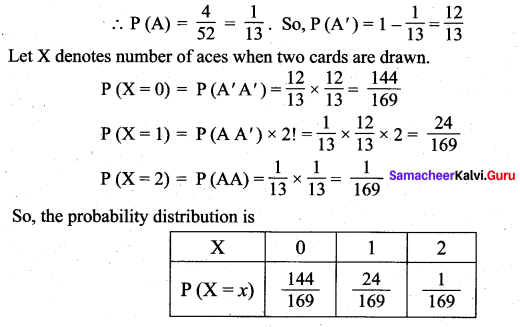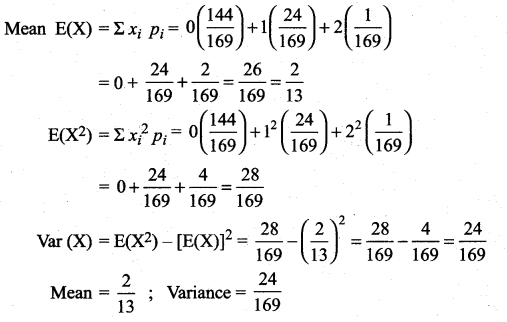## Tamilnadu Samacheer Kalvi 12th Maths Solutions Chapter 11 Probability Distributions Ex 11.4

Question 1.
For the random variable X with the given probability mass function as below, find the mean and variance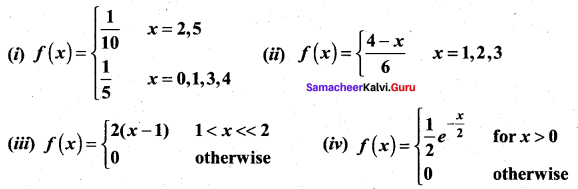Solution: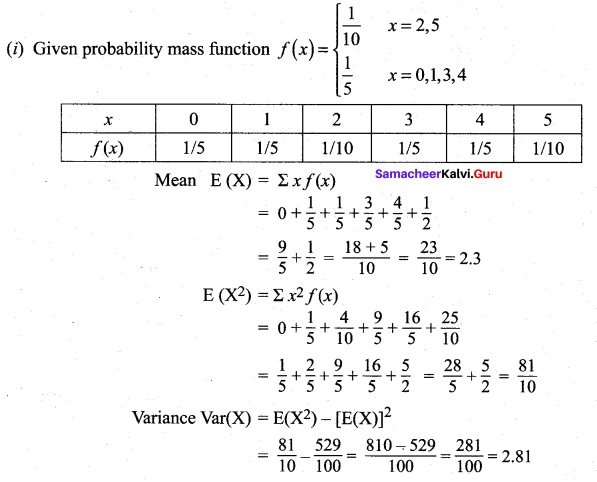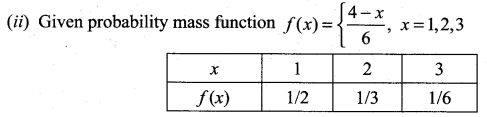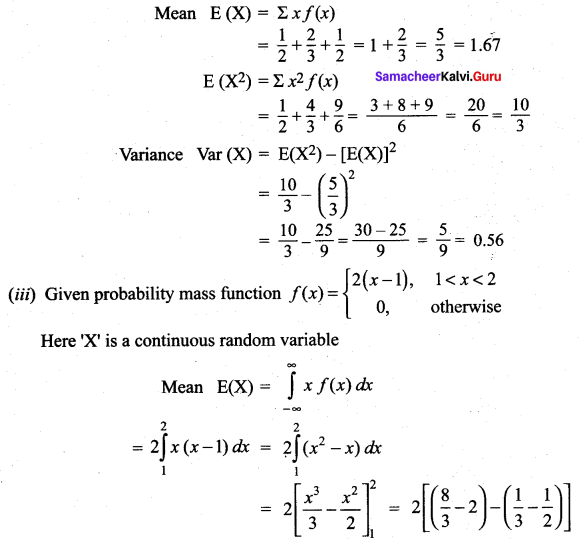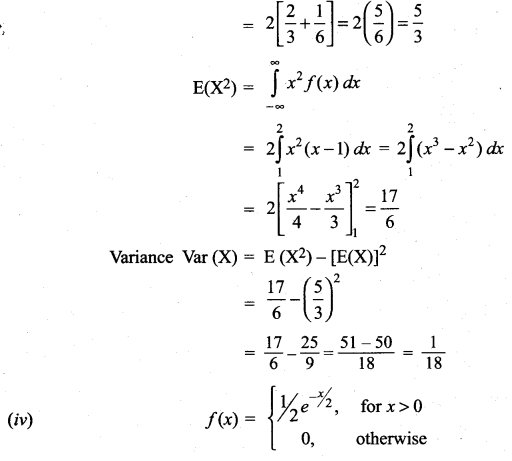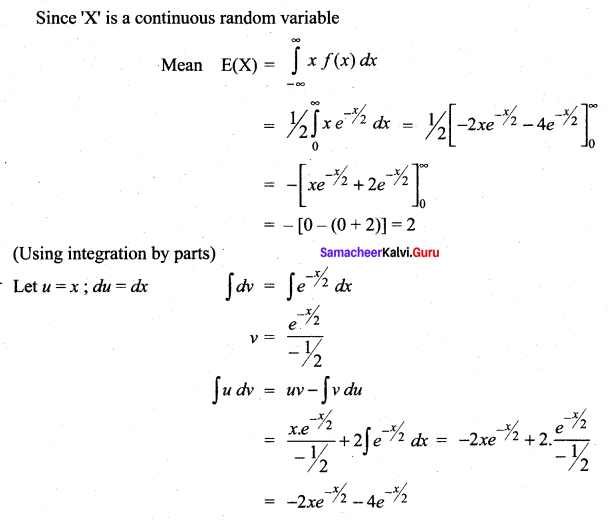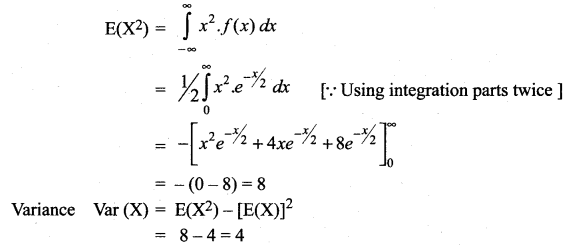Question 2.
Two balls are drawn in succession without replacement from an urn containing four red balls and three black balls. Let X be the possible outcomes drawing red balls. Find the probability mass function and mean for X.
Solution:
Number of Red balls = 4
Number of Black balls = 3
Total number of balls = 7
Given : two balls are drawn in succession without replacement.
Let ‘X’ be the number of red balls and ‘X’ can take the values 0, 1 and 2.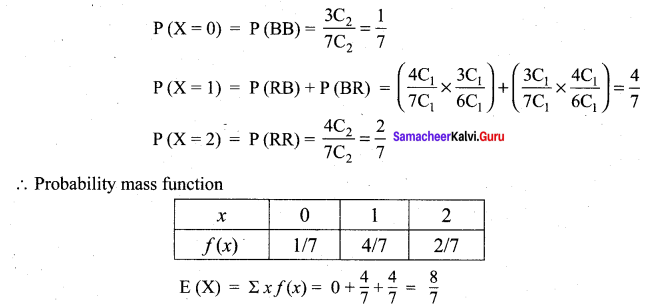Question 3.
If µ and σ2 are the mean and variance of the discrete random variable X, and E (X + 3) = 10 and E(X + 3)2 = 116, find µ and σ2.
Solution:
Mean = µ,
Variance = σ2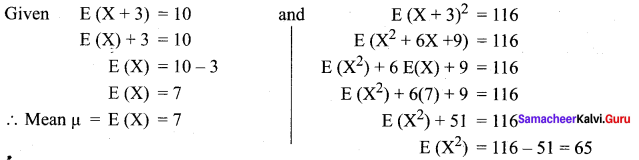Variance Var (X) = E (X2) — [E(X)]2
65 – 49 = 16 = σ2
∴ µ = 7 and σ2 = 16Question 4.
Four fair coins are tossed once. Find the probability mass function, mean and variance for number of heads occurred.
Solution:
Let ‘X’ be the number of heads occurred when four coins are tossed once. Hence ‘X’ can take the values 0, 1, 2, 3 and 4.
When 4 coins are tossed, the sample space is,
S = {HHHH, HHHT, HHTH, HHTT, HTHH, HTHT, HTTH, HTTT, THHH, THHT, THTH, THTT, TTHH, TTHT, TTTH, TTTT}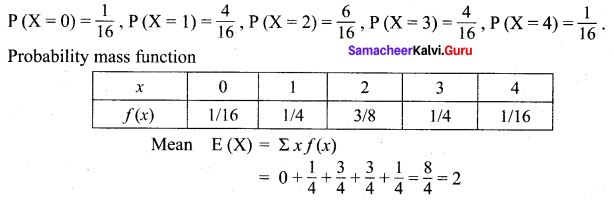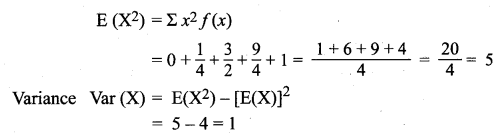Question 5.
A commuter train arrives punctually at a station every half hour. Each morning, a student leaves his house to the train station. Let X denote the amount of time, in minutes, that the student waits for the train from the time he reaches the train station. It is known that the p.d.f. of X is.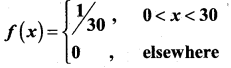Obtain and interpret the expected value of the random variable X.
Solution: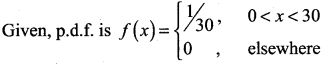‘X’ is a continuous random variable.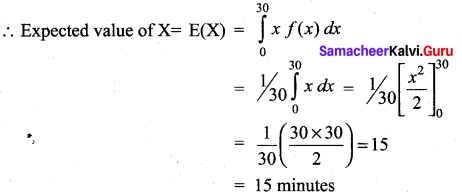Question 6.
The time to failure in thousands of hours of an electronic equipment used in a manufactured computer has the density function.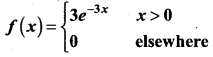Find the expected life of this electronic equipment.
Solution: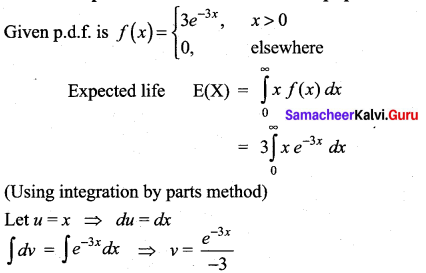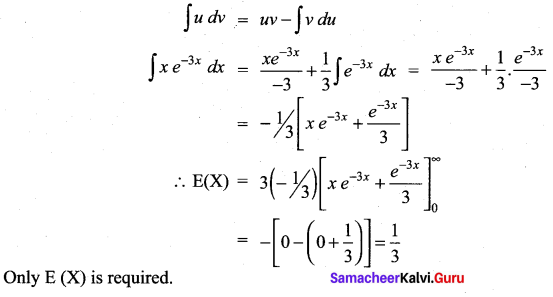Question 7.
The probability density function of the random variable X is given by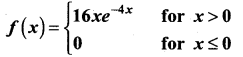Find the mean and variance of X.
Solution: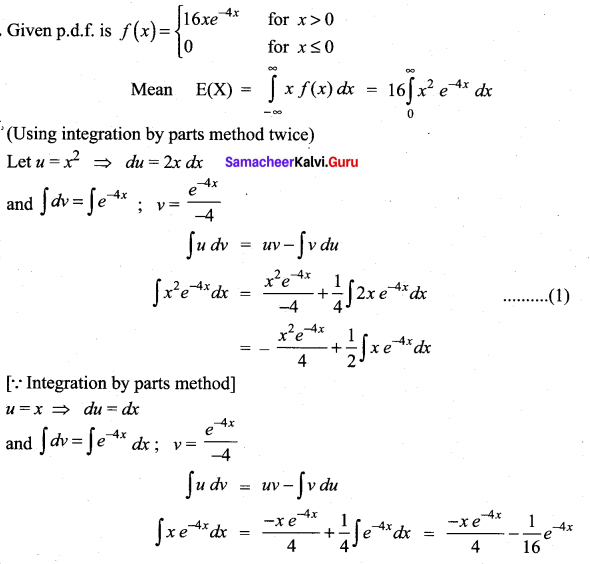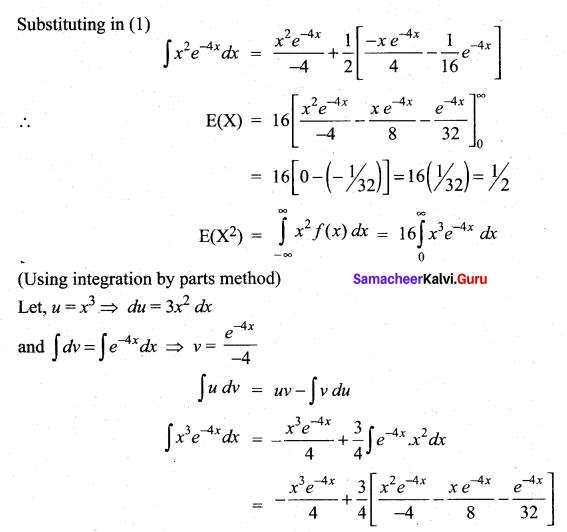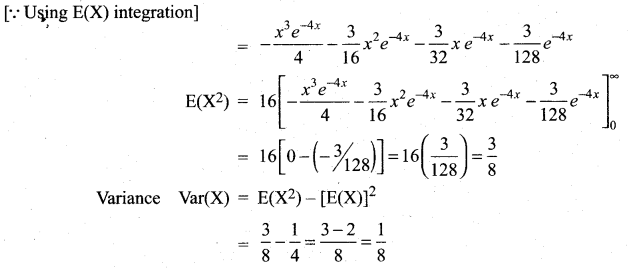Question 8.
A lottery with 600 tickets gives one prize of ₹ 200, four prizes of ₹ 100, and six prizes of ₹ 50. If the ticket costs is ₹ 2, find the expected winning amount of a ticket.
Solution:
Given, total number of tickets = 600
Prizes to be given : One prize of Rs. 200
Four prizes of Rs. 100
Six prizes of Rs. 50
Let ‘X’ be the random variable denotes the winning amount and it can take the values 200, 100 and 50.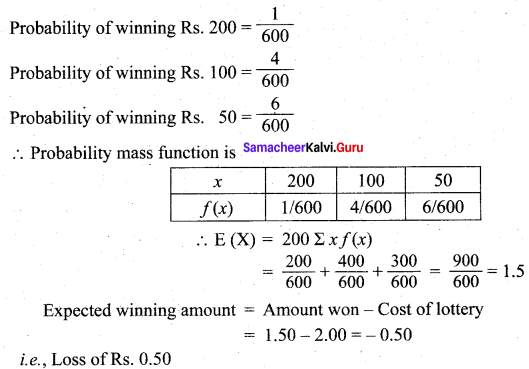### Samacheer Kalvi 12th Maths Solutions Chapter 11 Probability Distributions Ex 11.4 Additional Problems

Question 1.
The probability of success of an event is p and that of failure is q. Find the expected number of trials to get a first success.
Solution:
Let X be the random variable denoting ‘Number of trials to get a first success’. The success can occur in the 1st trial. ∴ The probability of success in the 1st trial is p. The success in the 2nd trial means failure in the 1st trial. ∴ Probability is qp.
Success in the 3rd trial means failure in the first two trials. Probability of success in the 3rd trial is q2p. As it goes on, the success may occur in the nth trial which mean the first (n – 1) trials are failures. probability = qn – 1p.
∴ The probability distribution is as follows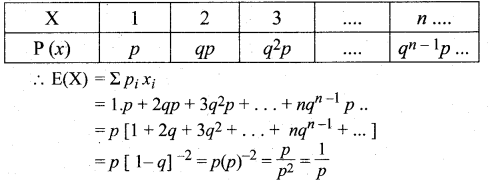Question 2.
An urn contains 4 white and 3 Red balls. Find the probability distribution of the number of red balls in three draws when a ball is drawn at random with replacement. Also find its mean and variance.
Solution:
The required probability distribution is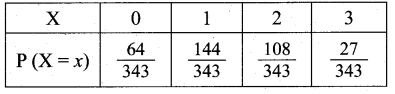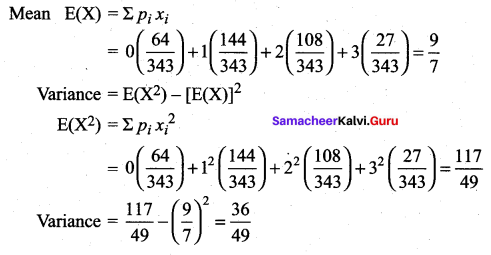Question 3.
Find the mean and variance of the distribution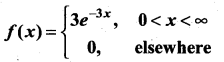Solution: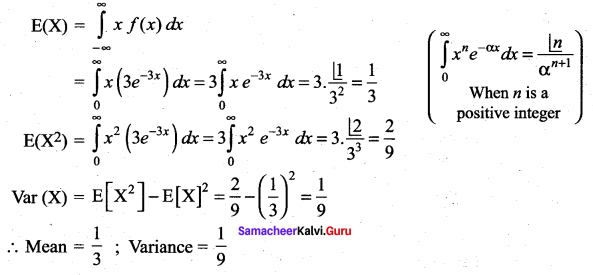Question 4.
Two cards are drawn with replacement from a well shuffled deck of 52 cards. Find the mean and variance for the number of aces.
Solution:
n (S) = 52
Number of aces = n (A) = 4